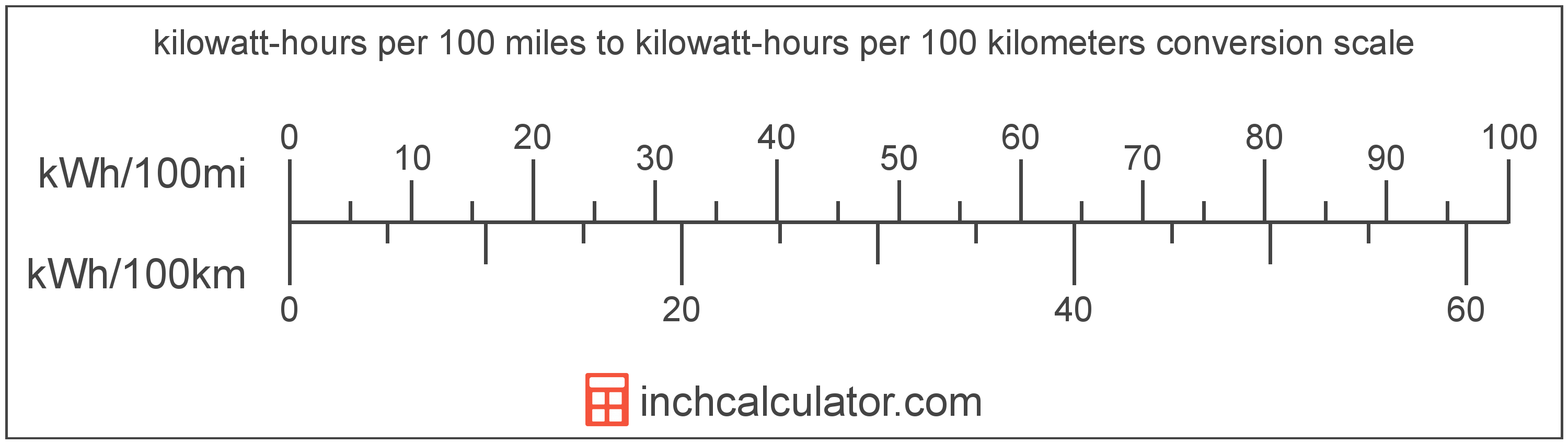# Kilowatt-Hours per 100 Miles to Kilowatt-Hours per 100 Kilometers Converter (KWh/100mi to KWh/100km)

Enter the electric car efficiency in kilowatt-hours per 100 miles below to get the value converted to kilowatt-hours per 100 kilometers.

Results in Kilowatt-Hours per 100 Kilometers:1 kWh/100mi = 0.621371 kWh/100km

Do you want to convert kWh/100km to kWh/100mi?

## How to Convert Kilowatt-Hours per 100 Miles to Kilowatt-Hours per 100 Kilometers

Convert kilowatt-hours per 100 miles to kilowatt-hours per 100 kilometers with this simple formula:

kWh/100km = kWh/100mi × 0.62137119223733

Insert the kWh/100mi efficiency measurement in the formula and then solve to find the result.

For example, let's convert 50 kWh/100mi to kWh/100km:

50 kWh/100mi = ( 50 × 0.62137119223733 ) = 31.06856 kWh/100kmKilowatt-hours per 100 miles and kilowatt-hours per 100 kilometers are both units used to measure electric car efficiency. Keep reading to learn more about each unit of measure.

## What Are Kilowatt-Hours per 100 Miles?

Kilowatt-hours per 100 miles are a measure of electric vehicle efficiency measuring the kilowatt-hours of energy required to travel 100 miles.

The kilowatt-hour per 100 miles is a US customary unit of electric car efficiency. Kilowatt-hours per 100 miles can be abbreviated as kWh/100mi; for example, 1 kilowatt-hour per 100 miles can be written as 1 kWh/100mi.

In the expressions of units, the slash, or solidus (/), is used to express a change in one or more units relative to a change in one or more other units.

## What Are Kilowatt-Hours per 100 Kilometers?

Kilowatt-hours per 100 kilometers are a measure of electric vehicle energy efficiency equal to the kilowatt-hours of energy needed for a vehicle to travel 100 kilometers.

The kilowatt-hour per 100 kilometers is an SI unit of electric car efficiency in the metric system. Kilowatt-hours per 100 kilometers can be abbreviated as kWh/100km; for example, 1 kilowatt-hour per 100 kilometers can be written as 1 kWh/100km.

## Kilowatt-Hour per 100 Miles to Kilowatt-Hour per 100 Kilometers Conversion Table

Table showing various kilowatt-hour per 100 miles measurements converted to kilowatt-hours per 100 kilometers.
Kilowatt-hours Per 100 Miles Kilowatt-hours Per 100 Kilometers
1 kWh/100mi 0.621371 kWh/100km
2 kWh/100mi 1.2427 kWh/100km
3 kWh/100mi 1.8641 kWh/100km
4 kWh/100mi 2.4855 kWh/100km
5 kWh/100mi 3.1069 kWh/100km
6 kWh/100mi 3.7282 kWh/100km
7 kWh/100mi 4.3496 kWh/100km
8 kWh/100mi 4.971 kWh/100km
9 kWh/100mi 5.5923 kWh/100km
10 kWh/100mi 6.2137 kWh/100km
11 kWh/100mi 6.8351 kWh/100km
12 kWh/100mi 7.4565 kWh/100km
13 kWh/100mi 8.0778 kWh/100km
14 kWh/100mi 8.6992 kWh/100km
15 kWh/100mi 9.3206 kWh/100km
16 kWh/100mi 9.9419 kWh/100km
17 kWh/100mi 10.56 kWh/100km
18 kWh/100mi 11.18 kWh/100km
19 kWh/100mi 11.81 kWh/100km
20 kWh/100mi 12.43 kWh/100km
21 kWh/100mi 13.05 kWh/100km
22 kWh/100mi 13.67 kWh/100km
23 kWh/100mi 14.29 kWh/100km
24 kWh/100mi 14.91 kWh/100km
25 kWh/100mi 15.53 kWh/100km
26 kWh/100mi 16.16 kWh/100km
27 kWh/100mi 16.78 kWh/100km
28 kWh/100mi 17.4 kWh/100km
29 kWh/100mi 18.02 kWh/100km
30 kWh/100mi 18.64 kWh/100km
31 kWh/100mi 19.26 kWh/100km
32 kWh/100mi 19.88 kWh/100km
33 kWh/100mi 20.51 kWh/100km
34 kWh/100mi 21.13 kWh/100km
35 kWh/100mi 21.75 kWh/100km
36 kWh/100mi 22.37 kWh/100km
37 kWh/100mi 22.99 kWh/100km
38 kWh/100mi 23.61 kWh/100km
39 kWh/100mi 24.23 kWh/100km
40 kWh/100mi 24.85 kWh/100km